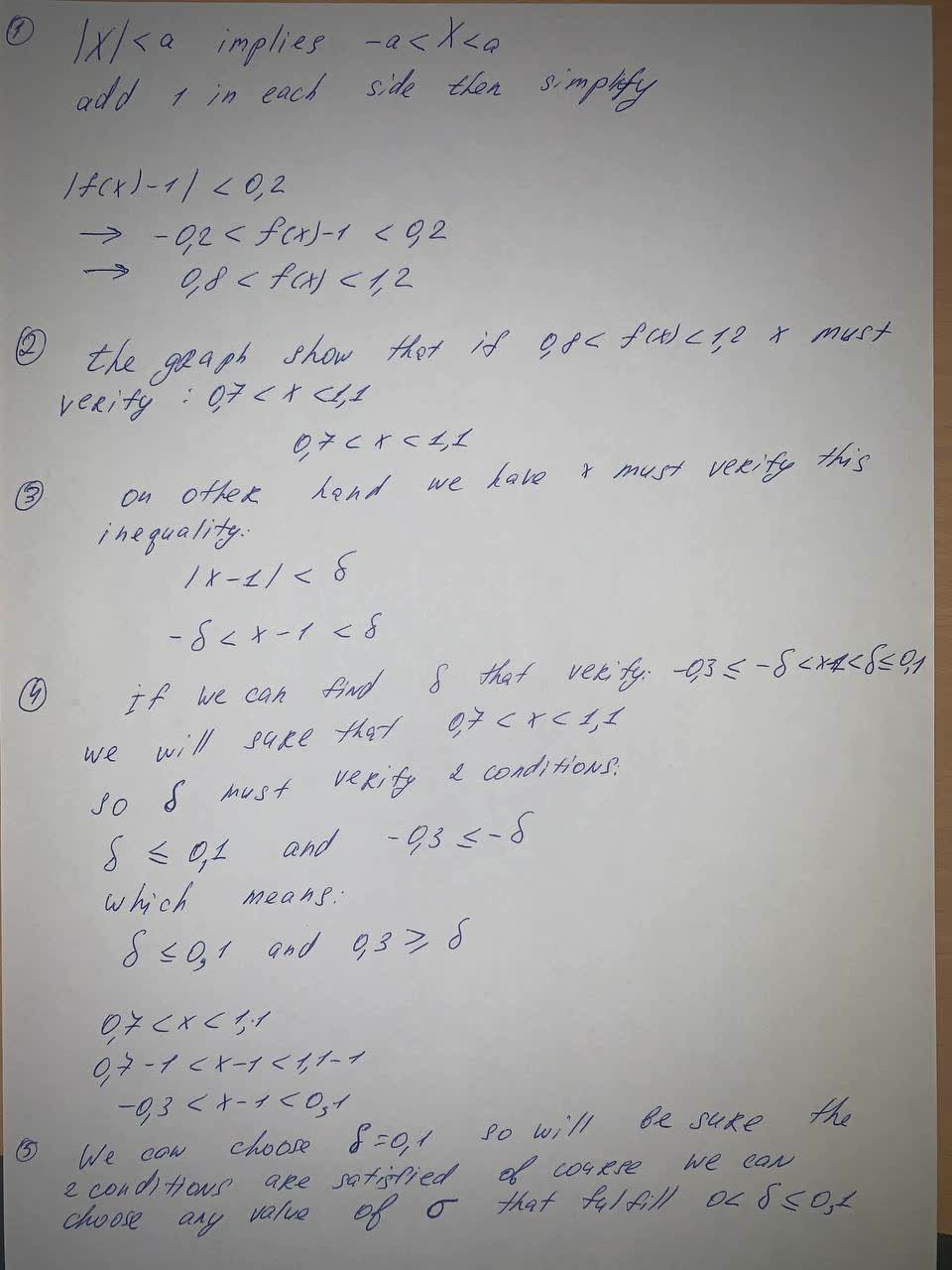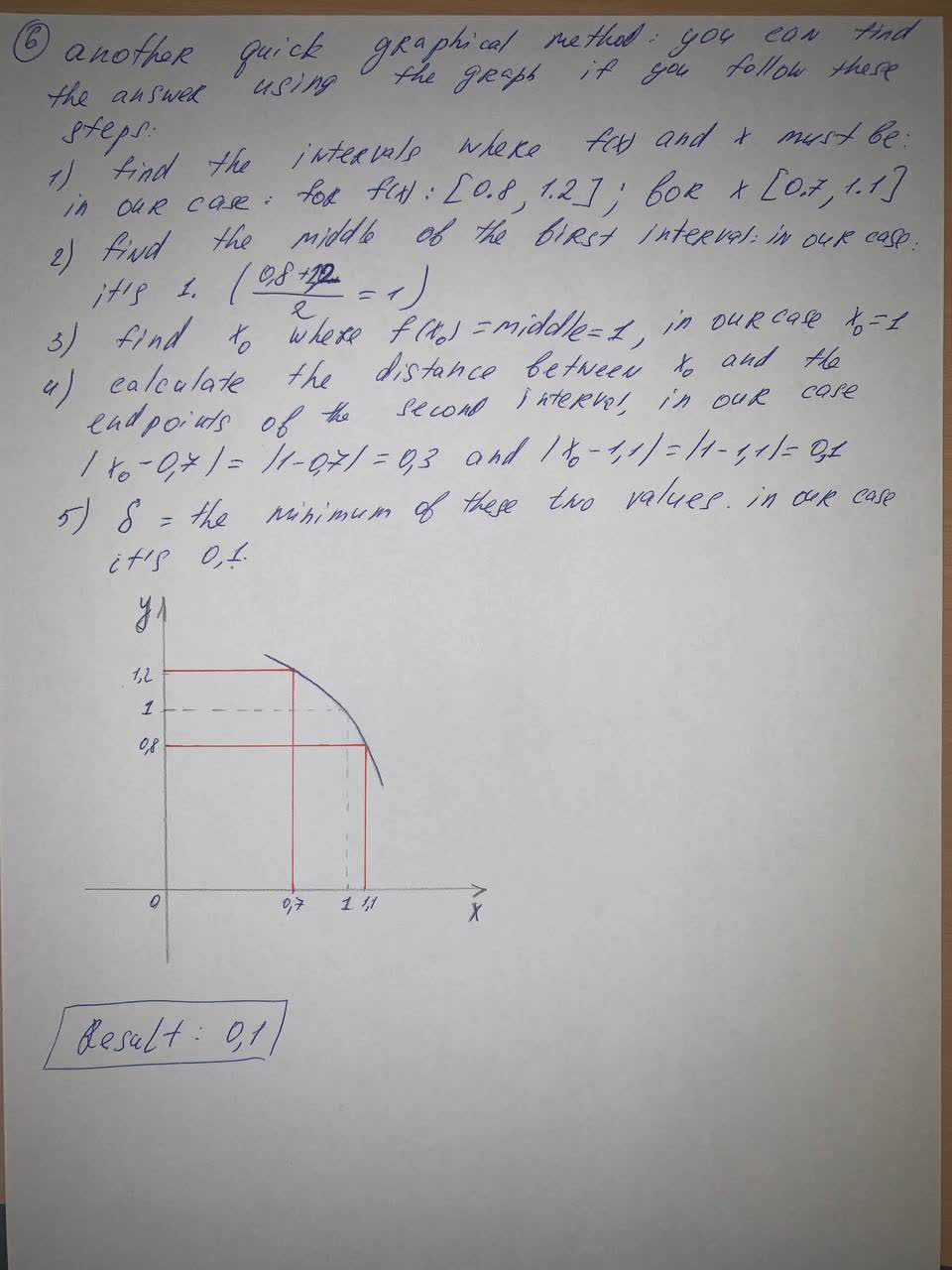# Use the given graph off to find a number \deltaglamrockqueen7 2021-08-21 Answered

Use the given graph off to find a number $$\displaystyle\delta$$ such that if $$|x-1| < \delta \text{ then } |f(x)-1| <0.2$$

• Questions are typically answered in as fast as 30 minutes

### Plainmath recommends

• Get a detailed answer even on the hardest topics.
• Ask an expert for a step-by-step guidance to learn to do it yourself.saiyansruleA
To find a number: